Circular Motion and Satellite Motion - Lesson 3 - Universal Gravitation

# The Apple, the Moon, and the Inverse Square Law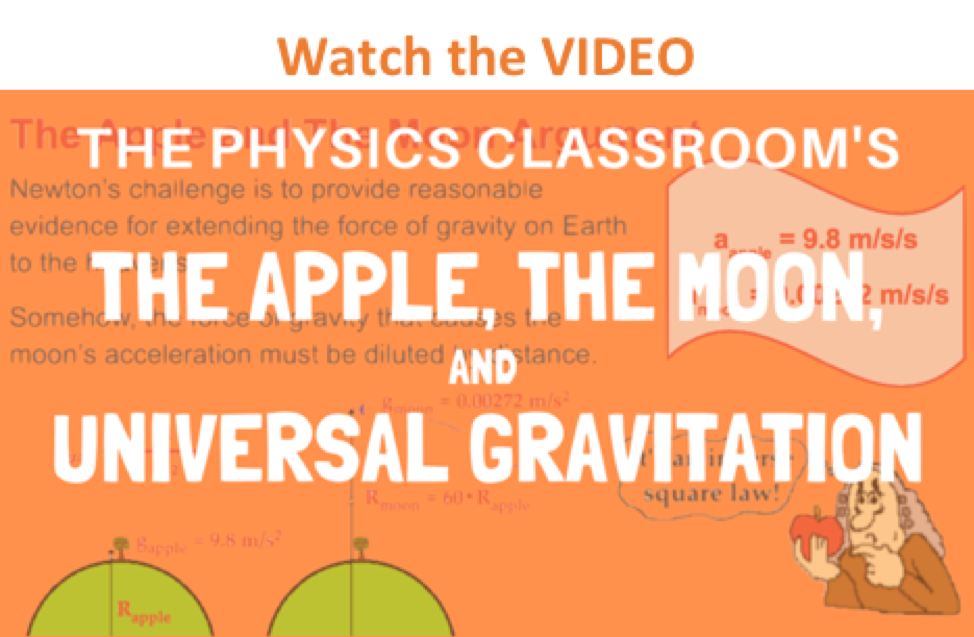In the early 1600's, German mathematician and astronomer Johannes Kepler mathematically analyzed known astronomical data in order to develop three laws to describe the motion of planets about the sun. Kepler's three laws emerged from the analysis of data carefully collected over a span of several years by his Danish predecessor and teacher, Tycho Brahe. Kepler's three laws of planetary motion can be briefly described as follows:

• The paths of the planets about the sun are elliptical in shape, with the center of the sun being located at one focus. (The Law of Ellipses)
• An imaginary line drawn from the center of the sun to the center of the planet will sweep out equal areas in equal intervals of time. (The Law of Equal Areas)
• The ratio of the squares of the periods of any two planets is equal to the ratio of the cubes of their average distances from the sun. (The Law of Harmonies)

(Further discussion of these three laws is given in Lesson 4.)

While Kepler's laws provided a suitable framework for describing the motion and paths of planets about the sun, there was no accepted explanation for why such paths existed. The cause for how the planets moved as they did was never stated. Kepler could only suggest that there was some sort of interaction between the sun and the planets that provided the driving force for the planet's motion. To Kepler, the planets were somehow "magnetically" driven by the sun to orbit in their elliptical trajectories. There was however no interaction between the planets themselves.

Newton was troubled by the lack of explanation for the planet's orbits. To Newton, there must be some cause for such elliptical motion. Even more troubling was the circular motion of the moon about the earth. Newton knew that there must be some sort of force that governed the heavens; for the motion of the moon in a circular path and of the planets in an elliptical path required that there be an inward component of force. Circular and elliptical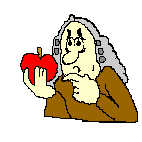motion were clearly departures from the inertial paths (straight-line) of objects. And as such, these celestial motions required a cause in the form of an unbalanced force. As learned in Lesson 1, circular motion (as well as elliptical motion) requires a centripetal force. The nature of such a force - its cause and its origin - bothered Newton for some time and was the fuel for much mental pondering. And according to legend, a breakthrough came at age 24 in an apple orchard in England. Newton never wrote of such an event, yet it is often claimed that the notion of gravity as the cause of all heavenly motion was instigated when he was struck in the head by an apple while lying under a tree in an orchard in England. Whether it is a myth or a reality, the fact is certain that it was Newton's ability to relate the cause for heavenly motion (the orbit of the moon about the earth) to the cause for Earthly motion (the falling of an apple to the Earth) that led him to his notion of universal gravitation.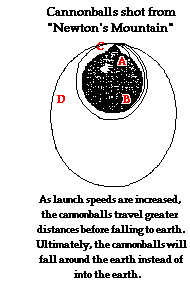### Newton's Mountain Thought Experiment

A survey of Newton's writings reveals an illustration similar to the one shown at the right. The illustration was accompanied by an extensive discussion of the motion of the moon as a projectile. Newton's reasoning proceeded as follows. Suppose a cannonball is fired horizontally from a very high mountain in a region devoid of air resistance. In the absence of gravity, the cannonball would travel in a straight-line, tangential path. Yet in the presence of gravity, the cannonball would drop below this straight-line path and eventually fall to Earth (as in path A). Now suppose that the cannonball is fired horizontally again, yet with a greater speed. In this case, the cannonball would still fall below its straight-line tangential path and eventually drop to earth. Only this time, the cannonball would travel further before striking the ground (as in path B). Now suppose that there is a speed at which the cannonball could be fired such that the trajectory of the falling cannonball matched the curvature of the earth. If such a speed could be obtained, then the cannonball would fall around the earth instead of into it. The cannonball would fall towards the Earth without ever colliding into it and subsequently become a satellite orbiting in circular motion (as in path C). And then at even greater launch speeds, a cannonball would once more orbit the earth, but in an elliptical path (as in path D). The motion of the cannonball orbiting to the earth under the influence of gravity is analogous to the motion of the moon orbiting the Earth. And if the orbiting moon can be compared to the falling cannonball, it can even be compared to a falling apple. The same force that causes objects on Earth to fall to the earth also causes objects in the heavens to move along their circular and elliptical paths. Quite amazingly, the laws of mechanics that govern the motions of objects on Earth also govern the movement of objects in the heavens.### Newton's Argument for Gravity Being Universal

Of course, Newton's dilemma was to provide reasonable evidence for the extension of the force of gravity from earth to the heavens. The key to this extension demanded that he be able to show how the affect of gravity is diluted with distance. It was known at the time, that the force of gravity causes earthbound objects (such as falling apples) to accelerate towards the earth at a rate of 9.8 m/s2. And it was also known that the moon accelerated towards the earth at a rate of 0.00272 m/s2. If the same force that causes the acceleration of the apple to the earth also causes the acceleration of the moon towards the earth, then there must be a plausible explanation for why the acceleration of the moon is so much smaller than the acceleration of the apple. What is it about the force of gravity that causes the more distant moon to accelerate at a rate of acceleration that is approximately 1/3600-th the acceleration of the apple?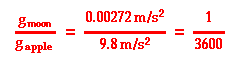Newton knew that the force of gravity must somehow be "diluted" by distance. But how? What mathematical reality is intrinsic to the force of gravity that causes it to be inversely dependent upon the distance between the objects?

The riddle is solved by a comparison of the distance from the apple to the center of the earth with the distance from the moon to the center of the earth. The moon in its orbit about the earth is approximately 60 times further from the earth's center than the apple is. The mathematical relationship becomes clear. The force of gravity between the earth and any object is inversely proportional to the square of the distance that separates that object from the earth's center. The moon, being 60 times further away than the apple, experiences a force of gravity that is 1/(60)2 times that of the apple. The force of gravity follows an inverse square law.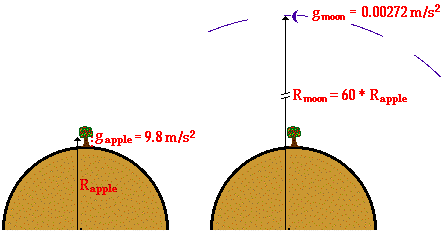The relationship between the force of gravity (Fgrav) between the earth and any other object and the distance that separates their centers (d) can be expressed by the following relationship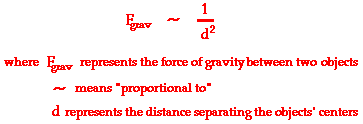Since the distance d is in the denominator of this relationship, it can be said that the force of gravity is inversely related to the distance. And since the distance is raised to the second power, it can be said that the force of gravity is inversely related to the square of the distance. This mathematical relationship is sometimes referred to as an inverse square law since one quantity depends inversely upon the square of the other quantity. The inverse square relation between the force of gravity and the distance of separation provided sufficient evidence for Newton's explanation of why gravity can be credited as the cause of both the falling apple's acceleration and the orbiting moon's acceleration.

### Using Equations as a Guide to Thinking

The inverse square law proposed by Newton suggests that the force of gravity acting between any two objects is inversely proportional to the square of the separation distance between the object's centers. Altering the separation distance (d) results in an alteration in the force of gravity acting between the objects. Since the two quantities are inversely proportional, an increase in one quantity results in a decrease in the value of the other quantity. That is, an increase in the separation distance causes a decrease in the force of gravity and a decrease in the separation distance causes an increase in the force of gravity. Furthermore,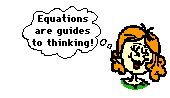the factor by which the force of gravity is changed is the square of the factor by which the separation distance is changed. So if the separation distance is doubled (increased by a factor of 2), then the force of gravity is decreased by a factor of four (2 raised to the second power). And if the separation distance is tripled (increased by a factor of 3), then the force of gravity is decreased by a factor of nine (3 raised to the second power). Thinking of the force-distance relationship in this way involves using a mathematical relationship as a guide to thinking about how an alteration in one variable affects the other variable. Equations can be more than recipes for algebraic problem solving; they can be guides to thinking. Check your understanding of the inverse square law as a guide to thinking by answering the following questions below. When finished, click the button to check your answers.

### We Would Like to Suggest ...Sometimes it isn't enough to just read about it. You have to interact with it! And that's exactly what you do when you use one of The Physics Classroom's Interactives. We would like to suggest that you combine the reading of this page with the use of our Gravitation Interactive. You can find it in the Physics Interactives section of our website. The Gravitation Interactive allows a learner to interactively explore the inverse square law of gravitation.

1 . Suppose that two objects attract each other with a gravitational force of 16 units. If the distance between the two objects is doubled, what is the new force of attraction between the two objects?

2. Suppose that two objects attract each other with a gravitational force of 16 units. If the distance between the two objects is tripled, then what is the new force of attraction between the two objects?

3. Suppose that two objects attract each other with a gravitational force of 16 units. If the distance between the two objects is reduced in half, then what is the new force of attraction between the two objects?

4. Suppose that two objects attract each other with a gravitational force of 16 units. If the distance between the two objects is reduced by a factor of 5, then what is the new force of attraction between the two objects?

5. Having recently completed his first Physics course, Noah Formula has devised a new business plan based on his teacher's Physics for Better Living theme. Noah learned that objects weigh different amounts at different distances from Earth's center. His plan involves buying gold by the weight at one altitude and then selling it at another altitude at the same price per weight. Should Noah buy at a high altitude and sell at a low altitude or vice versa?

Next Section: